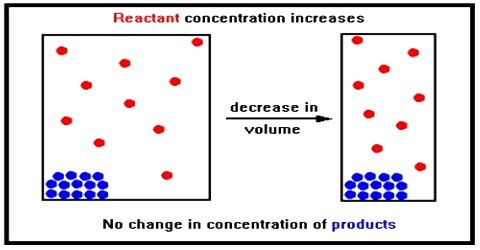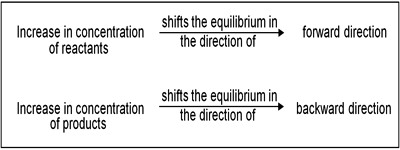# Effect of Change in Concentration on Chemical Equilibrium

Effect of change in concentration

If a chemical system at equilibrium experiences a change in concentration, temperature, volume, or partial pressure, then the equilibrium shifts to counteract the imposed change. Let us consider the equilibrium position for the dissociation of phosphorous pentachloride to phosphorous trichloride and chlorine:

PCl5 (g) ↔ PCl3 (g) + Cl2 (g)

If to the system at equilibrium some chlorine is added the concentration of chlorine increases. According to Le Chatelier principle, the system tries to diminish the effect of added chlorine. The effect of changes in the concentration of products and reactants in a reversible reaction can be predicted by Le Chatelier’s Principle. This is possible if the added chlorine is used up by combining with phosphorous trichloride until a new equilibrium is reached. This means that the equilibrium shifts to the left forming some phosphorous pentachloride.Same thing happens if some phosphorus trichloride added to the above equilibrium system. If, on the other hand, some phosphorous pentachloride is added to the equilibrium system the equilibrium will shift to the right.

The same conclusion can be arrival at by using the value of the equilibrium constant. At a given temperature the equilibrium constant for a particular reaction has a definite value even though one may start with different initial concentrations of either the reactants or products. The value of the equilibrium constant will not change if to the system at equilibrium some additional quantity of either the reactant or product is added. Considering the above equilibrium the equilibrium constant, KC is –

KC = [PCl3] [Cl2] / [PCl5]

If to the system at equilibrium some chlorine is added the concentration of chlorine increases. As the value of KC remains constant the denominator must increase. This is achieved if some chlorine combines with phosphorus trichloride to form phosphorus pentachloride, i.e., if the equilibrium shifts to the left. This is in accordance with Le Chatelier principle.

Similarly, if some phosphorus pentachloride is added to this system at equilibrium, part of it dissociates so that by adjustment of concentrations the value of KC is maintained constant.